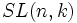# Generating set of a group

## Definition

### Symbol-free definition

A subset of a group is termed a generating set if it satisfies the following equivalent conditions:

• Every element of the group can be expressed as a word in terms of the elements of this subset, i.e., it can be expressed using the elements of the subset by means of the group operations of multiplication and inversion. (note that if the subset is a symmetric subset, i.e., it contains the identity and is closed under taking inverses, then every element of the group must be a product of elements in the subset. Symmetric subsets arise, for instance, when we take a union of subgroups).
• There is no proper subgroup of the group containing this subset
• There is a surjective map from a free group on that many generators to the given group, that sends the generators of the free group to the elements of this generating set.

The elements of the generating set are termed generators (the term is best used collectively for the generating set, rather than for the elements in isolation).

### Definition with symbols

A subset$S$ of a group$G$ is termed a generating set if it satisfies the following equivalent conditions:

• For any element$g \in G$, we can write:$g = a_1a_2 \ldots a_n$

where for each$a_i$, either$a_i \in S$ or$a_i^{-1} \in S$ (here, the$a_i$s are not necessarily distinct). In the situation where$S$ is a symmetric subset (i.e.$a_i \in S \implies a_i^{-1} \in S$) we do not need to throw in inverses. This happens, for instance, when$S$ is a union of subgroups of$G$.

• If$H$ is a proper subgroup of$G$ (i.e.$H$ is a subgroup of$G$ that is not equal to the whole of$G$), then$H$ cannot contain$S$.
• Consider the natural map from the free group on as many generators as elements of$S$, to the group$G$, which maps the freely generating set to the elements of$S$. This gives a surjective homomorphism from the free group, to$G$.

### Equivalence of definitions

Further information: Equivalence of definitions of generating set

## Examples

### Extreme examples

• The set of all elements of a group is a generating set for the group. It is also the largest possible generating set.
• The set of all non-identity elements of a group is a generating set for the group.
• If$S$ is a subset of a group$G$ such that every element of$G$ is a power of some element of$S$, then$S$ is a generating set for$G$.

### Some examples in abelian groups

• In the group of integers under addition, the singleton set$\{ 1 \}$ is a generating set. This is because every integer can be written as a sum of$1$s or$-1$s.
• In the group of integers under addition, the two-element set$\{ 2,3 \}$ is a generating set. To see this, note that every integer can expressed as a sum of$1$s or$-1$s, and both$1$ and$-1$ can be expressed in terms of$2$ and$3$.
• In the group of rational numbers, the set of all unit fractions$1/n, n \in \mathbb{N}$, form a generating set.

### Some examples in non-abelian groups

• In the symmetric group on a finite set, the set of all transpositions is a generating set for the group. For full proof, refer: Transpositions generate the finitary symmetric group
• The special linear group$SL(n,k)$ over a field$k$ is generated by elementary matrices. For full proof, refer: Elementary matrices generate the special linear group

## Constructs

### Cayley graph

Further information: Cayley graph of a group

Given a generating set of a group, we can construct the Cayley graph of the group with respect to that generating set. This is a graph whose vertex set is the set of elements of the group and where there is an edge between two vertices whenever one can be taken to the other by left multiplying by a generator.

### Presentation

The generators of a group may not in general be independent. That is, there may be words in the generators that simplify to the identity in the given group. Such a word is termed a relation between the generators. Relations can be viewed as elements of the free group on symbols corresponding to the generators. Thus relations form a normal subgroup of the free group. Specifying a set of relations whose normal closure is this normal subgroup is what we call a presentation of a group.

## Types of generating sets

Type of generating set Meaning Property of being a group that has such a generating set
finite generating set The generating set is finite as a set finitely generated group
minimal generating set (also known as irredundant generating set) The generating set has no proper subset that is also a generating set minimally generated group
generating set of minimum size A generating set such that there is no generating set of smaller size. If finite, then this must also be a minimal generating set

It is not in general true that any two minimal generating sets of a group have the same size (see [[. However, two important related facts are true:

## Study of this notion

### Mathematical subject classification

Under the Mathematical subject classification, the study of this notion comes under the class: 20F05